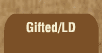Mathematics and Dyslexia

Tips for Learning the Times Tables

Tools for the Times Tables & Workbook

Home > Math > times tables practice > Eights (low, in order)

## The Eight Times Tables In Order - first set

Technically, you should know these already -- they've all been covered under the other tables. Make sure they're not rusty, though!

1. 8 x 0=
2. 8 x 1=
3. 8 x 2=
4. 8 x 3=
5. 8 x 4=
6. 8 x 5=
7. 5 x 8=
8. 4 x 8=
9. 3 x 8=
10. 2 x 8=
11. 1 x 8=
12. 2 x 8=
13. 3 x 8=
14. 4 x 8=
15. 3 x 8=